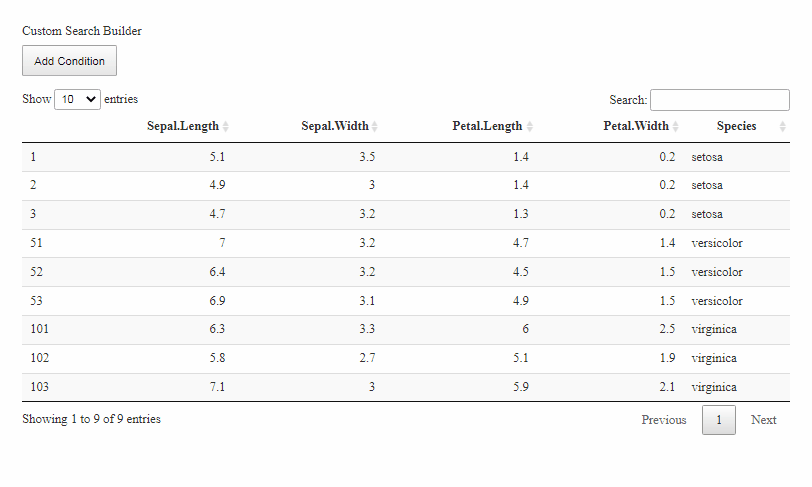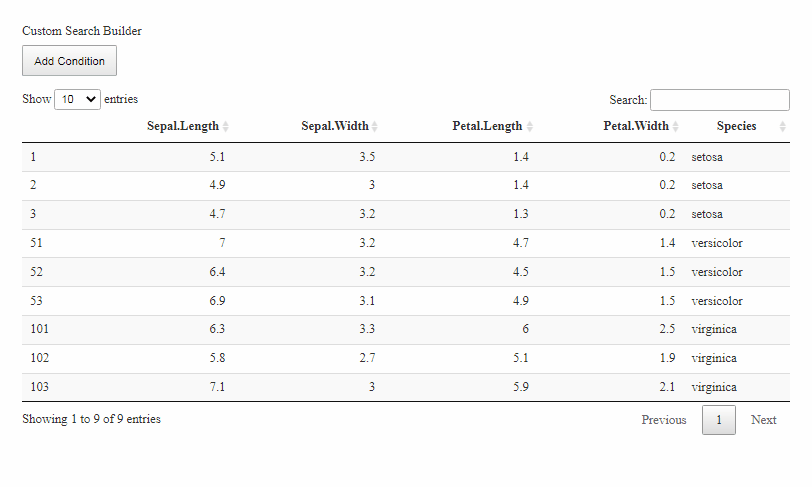# Search in a 'DT' table w/ or w/o a regular expression

Posted on July 3, 2022 by Stéphane Laurent

It is possible to search in a ‘DT’ table with a regular expression:

datatable(
data = dat,
options = list(
search = list(regex = TRUE)
)
)

But it could be desirable to have the possibility to search either with a regular expression or with an ordinary string.

The SearchBuilder extension allows to search in a table using numerous useful criteria, such as “contains”, “starts with”, “ends with”, etc:

library(DT)

dat <- iris[c(1:3, 51:53, 101:103), ]

datatable(
dat,
extensions = "SearchBuilder",
options = list(
dom = "Qlfrtip",
searchBuilder = TRUE
)
)In general, this is enough. But if really needed, it is possible to add a custom search criterion. Here is how to add a “matches regexp” criterion, to search with a regular expression:

datatable(
dat,
extensions = "SearchBuilder",
options = list(
dom = "Qlfrtip",
searchBuilder = list(
conditions = list(
string = list(
regexp = list(
conditionName = "Matches Regexp",
init = JS(
"function (that, fn, preDefined = null) {",
"  var el = $('<input/>').on('input', function() {", " fn(that, this);", " });", " if (preDefined !== null) {", "$(el).val(preDefined);",
"  }",
"  return el;",
"}"
),
inputValue = JS(
"function (el) {",
"  return $(el).val();", "}" ), isInputValid = JS( "function (el, that) {", " var regexp =$(el).val();",
"  var valid = true;",
"  try {",
"    new RegExp(regexp, 'g');",
"  } catch(e) {",
"    valid = false;",
"  }",
"  return valid;",
"}"
),
search = JS(
"function (value, regexp) {",
"  var reg = new RegExp(regexp, 'g');",
"  return reg.test(value);",
"}"
)
)
)
)
)
)
)